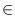Next: Sets of Nodes ( Up: Graphs and Related Data Previous: Two Dimensional Node Arrays   Contents   Index

# Two-Dimensional Node Maps ( node_map2 )

Definition

An instance of the data type node_map2<E> is a map2 for the pairs of nodes of a graph G, i.e., equivalent to map2 < node, node, E > (cf. Two-Dimensional Maps). It can be used as a dynamic variant of the data type nodematrix (cf. Two Dimensional Node Arrays).

#include < LEDA/graph/node_map2.h >

Creation

 node_map2 M introduces a variable M of type node_map2 and initializes it to the map2 with empty domain. node_map2 M(const graph_t& G) introduces a variable M of type node_map2 and initializes it with a mapping m from the set of all nodes of G into the set of variables of type E. The variables in the range of m are initialized by a call of the default constructor of type E. node_map2 M(const graph_t& G, E x) introduces a variable M of type node_map2 and initializes it with a mapping m from the set of all nodes of G into the set of variables of type E. The variables in the range of m are initialized with a copy of x.

Operations

 void M.init() makes M a node map2 with empty domain. void M.init(const graph_t& G) makes M to a mapping m from the set of all nodes of G into the set of variables of type E. The variables in the range of m are initialized by a call of the default constructor of type E. void M.init(const graph_t& G, E x) makes M to a mapping m from the set of all nodes of G into the set of variables of type E. The variables in the range of m are initialized with a copy of x. E& M(node v, node w) returns the variable M(v, w). bool M.defined(node v, node w) returns true if (v, w)dom(M) and false otherwise.

Implementation

Node maps are implemented by an efficient hashing method based on the internal numbering of the nodes. An access operation takes expected time O(1).Next: Sets of Nodes ( Up: Graphs and Related Data Previous: Two Dimensional Node Arrays   Contents   Index